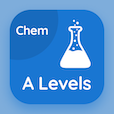Cambridge Online Courses (GCE)

A Level Physics Quizzes

A Level Physics Quiz PDF - Complete

# Electrical Potential Multiple Choice Questions p. 59

Study Electrical Potential multiple choice questions and answers, electrical potential quiz answers PDF 59 to study A Level Physics course online. AS Level Physics MCQ trivia questions, electrical potential Multiple Choice Questions (MCQ) for online college degrees. "Electrical Potential MCQ" PDF eBook: operational amplifier, simple harmonic motion gravitation, sinusoidal current, particles and waves modeling, electrical potential test prep for ACT practice test.

"If we move a positive charge to a positive plate, then potential energy of charge is" MCQ PDF: increased, decreased, remains constant, and dissipated for schools that offer online degrees. Learn as level physics questions and answers to improve problem solving skills for SAT practice test.

## Electrical Potential Questions and Answers MCQs

MCQ: If we move a positive charge to a positive plate, then potential energy of charge is

decreased
increased
remains constant
dissipated

MCQ: Plank's constant has units

J
s
J s-1
J s

MCQ: Graph of alternating current is a

cos wave
tan wave
curve
sine wave

MCQ: Stationary waves are also called

static waves
standing waves
progressive waves
All of the above

MCQ: Amplifier produces output with more

power only
voltage only
current only
power and voltage

### More Quizzes from A Level Physics Course